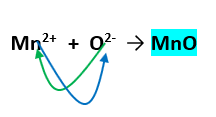# Problem: What is the empirical formula for manganese(II) oxide?

###### FREE Expert Solution

We are asked to determine what is the empirical formula for manganese(II) oxide.

Recall that the empirical formula is the simplest whole number ratio formula of a compound. On the other hand, the molecular formula is the unsimplified and actual formula of a compound.

To identify the empirical formula we need to check if the formula of a compound can be simplified further. This means we need to check if we can divide the formula by a whole number.

We’re being asked to determine the molecular formula of manganese(II) oxide. The rules for naming binary ionic compounds are as follows:

1. The metal keeps its name, and is named and written first.

– For transition metals, we use Roman numerals to denote its charge.

2. The nonmetal keeps its base name but has its ending change to –ide.

We can see that the metal in the ionic compound is manganese (Mn), which is a transition metal. This means it has many possible charges.

The nonmetal, oxide has a charge of -2 hence O2-manganese (Mn) therefore has a charge of +2, Mn2+1 Mn combines with 1 O to cancel the charges

84% (289 ratings)###### Problem Details

What is the empirical formula for manganese(II) oxide?